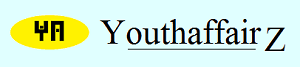﻿ Youthaffairz | Teaser: Grazing cowsJuly 2015

# Solution

Now, the area of the plot that can be grazed by any cow will depend upon the angle of the triangle at the corner to which it is tied and the length of the rope. For instance, if a cow is tied to a pole with a rope of length 7 metres and there are no boundaries to impede its movement, then it can graze all around and within a circle of radius 7 metres (thus, it can move through 360 degrees). However, if its movement is restricted and it can move only through 180 degrees, it will be able to graze only half of the plot; if its movement is restricted so that it can move through only 90 degrees, then it can graze only one-fourth of the plot. Thus, the angle and radius will determine how far it can graze.

In our case let the vertices of the triangle be A, B and C. Let the side opposite "A" be "a", the side opposite "B" be "b" and the side opposite "C" be "c".

The area of a circle is pi x r2 where pi = 22/7. Thus, the cow at "A" will be able to graze pi x r2/(360/A) area, cow at "B" will graze pi x r2/(360/B) and cow at "C" will graze pi x r2/(360/C).

Therefore the total area grazed by the three will be:

pi x r2/(360/A) + pi x r2/(360/B) + pi x r2/(360/C).

= (A x pi x r2)/360 + (B x pi x r2)/360 + (C x pi x r2)/360.

= [(pi x r2)(A + B + C)]/360.

But sum of the angles of a triangle is 180, so A + B + C = 180.

Therefore, the total area grazed by the three will be: pi x r2/2.

Therefore, the total area grazed by the three will be: 22/7 x 7 x 7 /2 (because radius = 7).

Therefore, the total area grazed by the three will be: 77 square metres.

Did I do it right, or did I get it all wrong?

Well, to find the total area grazed I did not have to take into account the length of the sides of the triangle or the actual values of the angles. But if I want to find the areas grazed by each cow, I think I will need to know the angles. I can find the angles using the Cosine Rule. We have marked the vertices of the triangle as A, B and C and the respective opposite sides are a, b and c.

According to the Cosine Rule: Cos A = (b2 + c2 - a2)/2bc.

Similarly, Cos B = (a2 + c2 - b2)/2ac, and

Cos C = (a2 + b2 - c2)/2ab.

In our case, a = 20, b = 30 and c = 40.

Therefore, Cos A = (900 + 1600 - 400)/2400 = 7/8 = 0.875. If Cos A = 0.875, then a trigonometric calculator tells me that A = 28.95 degrees (approx). Therefore, the cow at A will be able to graze pi x r2/(360/A) = 22/7 x 7 x 7 x 28.95/360 = 154 x 28.95/360 = 12.384 square metres.

Cos B = (a2 + c2 - b2)/2ac; so, Cos B = (400 + 1600 - 900)/1600 = 11/16 = 0.6875. If Cos B = 0.6875, then a trigonometric calculator tells me that B = 46.57 (approx). Therefore, the cow at B will be able to graze 154 x 46.57/360 = 19.92 square metres.

Cos C = (a2 + b2 - c2)/2ab; so, Cos C = (400 + 900 - 1600)/1200 = -3/12 = -1/4 = -0.25. If Cos C = -0.25, then a trigonometric calculator tells me that C = 104.48 degrees. Therefore, the cow at C will be able to 154 x 104.48/360 = 44.694 square metres.

I must have got the whole thing wrong. Do let me know whether I did it right.

• Union Public Service Commission - www.upsc.gov.in
• IIT-Kharagpur - www.iitkgp.ac.in
• Indian Statistical Institute - www.isical.ac.in
• Indian Institute of Technology Madras - www.iitm.ac.in
• Indian Institute of Management, Ahmedabad - www.iimahd.ernet.in
• Indian Institute of Mass Commission - www.iimc.nic.in
• IIT Bombay - www.iitb.ac.in